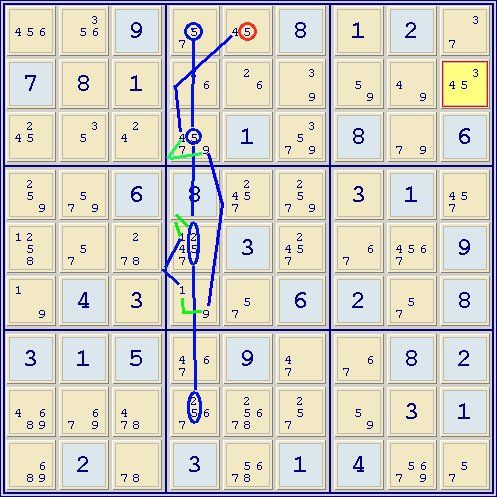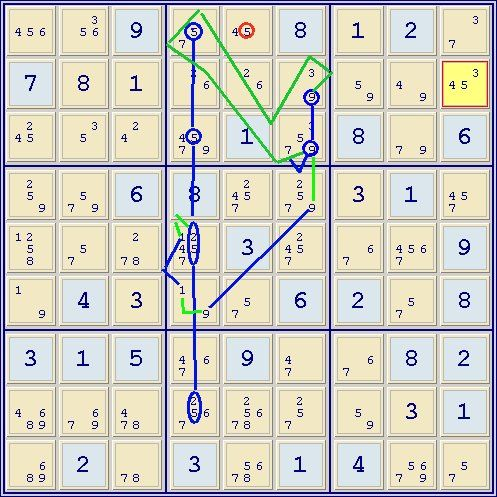Gurth from Dec 23, 2014

Post puzzles for others to solve here.

Gurth from Dec 23, 2014

Gurth introduced this ER=8.3 puzzle at the end of the following thread:
http://forum.enjoysudoku.com/december-23-2014-t32232.html
I don't know what the source of it was, but it is one of the more interesting puzzles that I've come across in a long time- so thanks to Gurth.

Code: Select all
`*-----------* |..9|..8|.2.| |7..|...|...| |...|.1.|8.6| |---+---+---| |..6|8..|.1.| |...|.3.|..9| |.4.|..6|2.8| |---+---+---| |315|.9.|.82| |...|...|..1| |.2.|3.1|4..| *-----------*`

Code: Select all
`*-----------------------------------------------------------------------------* | 1456    356     9       | 4567    4567    8       | 1357    2       3457    | | 7       3568    12348   | 24569   2456    23459   | 1359    3459    345     | | 245     35      234     | 24579   1       234579  | 8       34579   6       | |-------------------------+-------------------------+-------------------------| | 259     3579    6       | 8       2457    24579   | 357     1       3457    | | 1258    578     1278    | 12457   3       2457    | 567     4567    9       | | 159     4       137     | 1579    57      6       | 2       357     8       | |-------------------------+-------------------------+-------------------------| | 3       1       5       | 467     9       47      | 67      8       2       | | 4689    6789    478     | 2567    25678   257     | 35679   35679   1       | | 689     2       78      | 3       5678    1       | 4       5679    57      | *-----------------------------------------------------------------------------*`

I expect to have to use nets to solve puzzles of difficulty above around ER=8.5, but below that I like to try to avoid them so this puzzle was solved with relatively simple chains: No nets (ie. AAICs/Krakens) and not even patterns (ie. empty rectangle, broken wings/guardians etc.) that often require net-like chains. It's possible that the solution could be further optimized, but I decided to stick with the first run.

A puzzle like this doesn't die easily so each chain may lead to one or more basic methods. I prefer to show those below the numbered chain that gave rise to them rather than number them individually. Fwiw: I don't see almost-hidden sets used on this forum that much. They are very useful constructs; two of them are used here.

1. (1)r2c3=(1-9)r2c7=r8c7-r8c2=r4c2-r4c6=(9-1)r6c4=(1)r5c4 => -1r5c3
2. (7)r5c2=aHP(79-3)r48c2=(3)r6c3 => -7r6c3
3. (1)r2c3=(1-3)r6c3=r6c8-r8c8=(3-9)r8c7=(9)r2c7 => -1r2c7
=> singles: r2c3=1,r1c7=1,r2c2=8,r6c3=3
=> LCs: –6r1c45, -2r3c46 np(57)r6c58 -> -5r6c14,-7r6c4
=> grp skyscraper: (7)c4568r36 => -7r1c5
4. grp(9)r3c46=r3c8-r9c8=r9c1-r6c1=(9)r6c4 => -9r2c4
5. als(459=3)r2c789-r2c6=r3c6-(3=5)r3c2-als(5=79)r45c2-(9)r6c1=r6c4-als(9=457)r3c6,r1c45 => -45r2c456
=> np(26)r2c45 => -2r2c6
=> LCs: -45r1c9, -45r3c8
6. (3)r4c7=(3-4)r4c9=(4-6)r5c8=grp(6)r89c8-(6=7)r7c7 => -7r4c7
7. pausingchn(4=7)r7c6-(7=6)r7c7-r5c7=(6-4)r5c8=*(4)r4c9-r4c5=(4)r1c5 => -4r34c6
8. (3=5)r4c7-r6c8=r6c5-(4=5)r1c5-r4c5=(4)r4c9 => -3r4c9
=> singles: r4c7=3, r8c8=3
=> xy-wing: (79)r3c8[(59)r2c7,(57)r6c8] => -5r1c8,-5r5c7
=> np(67)r57c7 => -67r8c7
9. (7)r1c4=(7-3)r1c9=(3-4)r2c9=r4c9-r4c5=(4)r1c5 => -4r1c4
10. grp(5)r13c4=aHP(25-1)r58c4=(1-9)r6c4=(9-4)r3c4=(4)r1c5 => r1c5<>5=4 -> r4c9=4
=> nt(356)box1 => -5r3c1
=> nt(567)r5c278: -5r5c146, -7r5c346
=> LCs: -7r8c2, -5r9c8
11. (9=1)r6c4-als(1=24)r5c46-(2=8)r5c3-(8=7)r9c3-r9c9=r1c9-(7=9)r3c8 => -9r3c4

stte

Step 10 was a critical step and uses an almost-hidden pair. For those that might be interested:Below: Alternative end of step 10: grp(5)r13c4=aHP(25-1)r58c4=(1-9)r6c4=r4c6-als(9=357)r1c4,r23c6Last edited by DonM on Wed Jan 28, 2015 6:18 pm, edited 1 time in total.
DonM
2013 Supporter

Posts: 475
Joined: 13 January 2008

Re: Gurth from Dec 23, 2014

Glad you enjoyed the puzzle, DonM. I should have added a name to it - basically its name is 4cp, which is just the date of composition - 4=2014, c=Dec, p=25th. (I fight redundancy on all fronts!!I wish I could remember how I made it, usually they are original, not computer generated, but sometimes I make a puzzle by letting SS or SE create a puzzle, then stripping away redundant clues. My memory is short these days.
And there I was trying to solve this puzzle on one piece of paper! A rather vain hope, my picture actually looks pretty ghastly, in reducing the rating from 8.3 to 7.2. Whereas a picture like your first diagram is rather irresistible, and I enjoyed following it. I didn't follow the second pic as I thought the first one entirely adequate.
gurth

Posts: 358
Joined: 11 February 2006
Location: Cape Town, South Africa